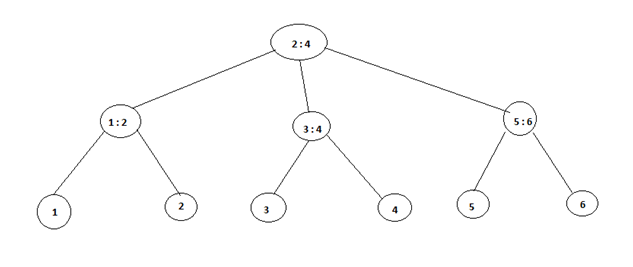# 2 – 3 Trees Algorithm

In this article, we will learn the concept of 2 – 3 trees with its algorithm.
Submitted by Shivangi Jain, on July 29, 2018

## 2 – 3 Trees

A 2 – 3 trees also known 3 – 2 trees is a tree in which each vertex, except leaf has 2 or 3 sons and one or two keys per node, and every path from the root to leaf is of the same length. The tree consisting of a single vertex is a 2 – 3 trees.

Let T be a 2 – 3 trees of height h. The number of vertices of T is between (2^h+1 - 1) and (3^h+1 - 1)/2, and the number of leaves is in between 2^h and 3^h.

Inserting a key K into a B tree T of height h is done in a single pass down the tree, requiring O (h) disk accesses. The CPU time required is O (th) = O (t log n). the B tree insert procedure uses B tree split child to guarantee that the recursion never descends to a full node.2 - 3 Trees

Algorithm:

```    1.	B tree insert (T, K)
2.	r = root [T]
3.	if n[r] = 2t – 1
4.	then s = ALLOCATE – NODE ()
5.	root [T] = s
6.	leaf [s] = FALSE
7.	n [s] = 0
8.	c1 [s] = r
9.	B – TREE – SPLIT – CHILD (s, 1, r)
10.	B – TREE – INSERT – NONFULL (s, k)
11.	Else
12.	B – TREE – INSERT – NONFULL (r, k)
```

The lines 4 to 10 deals with the case in which the root node r is full – the root is split and a new node s (having two children) becomes the root. Splitting the root is the only way to increase the height of a B tree. Unlike a binary search tree, a B tree increases in height at the top instead of at the bottom. Afterwards, the procedure finishes by calling B – TREE – INSERT – NONFULL to perform the insertion of key k in the tree rooted at the non-full root node. B – TREE – INSERT – NONFULL recurses as necessary down the tree, at all times guaranteeing that the node to which it recurses is not full by calling B – TREE- SPLIT – CHILD as necessary.

B – TREE – INSERT – NONFULL inserts a key K into the node x, which is assumed to be non-full when the procedure is called. The operation of B – TREE – INSERT and the recursive operation of B – TREE – INSERT – NONFULL guarantees that this assumption is true.

Algorithm:

```    1.	B – TREE – INSERT – NONFULL (x, k)
2.	 i = n [x]
3.	If leaf [x]
4.	Then while i>= 1 and k< key(i) [x]
5.	Do key(i+1)[x] = key (i) [x]
6.	i = i – 1
7.	key (i+1) [x] = k
8.	n[x] = n[x] + 1
9.	DISK_ WRITE (x)
10.	Else while i>= 1 and K < key (i)[x]
11.	Do i = i – 1
12.	i = i + 1
14.	If n [c(i)[x]] = 2t - 1
15.	Then B – TREE – SPLIT – CHILD (x, I, c(i)[x])
16.	If k> key (i)[x]
17.	Then i = i + 1
18.	B – TREE – INSERT – NONFULL (c(i)[x], k)
```

References: